# NCERT solutions for Mathematics Exemplar Class 9 chapter 8 - Quadrilaterals [Latest edition]

#### ChaptersExercise 8.1Exercise 8.2Exercise 8.3Exercise 8.4
Exercise 8.1 [Pages 73 - 75]

### NCERT solutions for Mathematics Exemplar Class 9 Chapter 8 Quadrilaterals Exercise 8.1 [Pages 73 - 75]

#### Choose the correct alternative:

Exercise 8.1 | Q 1 | Page 73

Three angles of a quadrilateral are 75º, 90º and 75º. The fourth angle is ______.

• 90º

• 95º

• 105º

• 120º

Exercise 8.1 | Q 2 | Page 73

A diagonal of a rectangle is inclined to one side of the rectangle at 25º. The acute angle between the diagonals is ______.

• 55º

• 50º

• 40º

• 25º

Exercise 8.1 | Q 3 | Page 73

ABCD is a rhombus such that ∠ACB = 40º. Then ∠ADB is ______.

• 40º

• 45º

• 50º

• 60º

Exercise 8.1 | Q 4 | Page 73

The quadrilateral formed by joining the mid-points of the sides of a quadrilateral PQRS, taken in order, is a rectangle, if ______.

• PQRS is a rectangle

• PQRS is a parallelogram

• Diagonals of PQRS are perpendicular

• Diagonals of PQRS are equal

Exercise 8.1 | Q 5 | Page 73

The quadrilateral formed by joining the mid-points of the sides of a quadrilateral PQRS, taken in order, is a rhombus, if ______.

• PQRS is a rhombus

• PQRS is a parallelogram

• Diagonals of PQRS are perpendicular

• Diagonals of PQRS are equal

Exercise 8.1 | Q 6 | Page 74

If angles A, B, C and D of the quadrilateral ABCD, taken in order, are in the ratio 3:7:6:4, then ABCD is a ______.

• Rhombus

• Parallelogram

• Trapezium

• Kite

Exercise 8.1 | Q 7 | Page 74

If bisectors of ∠A and ∠B of a quadrilateral ABCD intersect each other at P, of ∠B and ∠C at Q, of ∠C and ∠D at R and of ∠D and ∠A at S, then PQRS is a ______.

• Rectangle

• Rhombus

• Parallelogram

• Quadrilateral whose opposite angles are supplementary

Exercise 8.1 | Q 8 | Page 74

If APB and CQD are two parallel lines, then the bisectors of the angles APQ, BPQ, CQP and PQD form ______.

• A square

• A rhombus

• A rectangle

• Any other parallelogram

Exercise 8.1 | Q 9 | Page 74

The figure obtained by joining the mid-points of the sides of a rhombus, taken in order, is ______.

• A rhombus

• A rectangle

• A square

• Any parallelogram

Exercise 8.1 | Q 10 | Page 74

D and E are the mid-points of the sides AB and AC of ∆ABC and O is any point on side BC. O is joined to A. If P and Q are the mid-points of OB and OC respectively, then DEQP is ______.

• A square

• A rectangle

• A rhombus

• A parallelogram

Exercise 8.1 | Q 11 | Page 74

The figure formed by joining the mid-points of the sides of a quadrilateral ABCD, taken in order, is a square only if, ______.

• ABCD is a rhombus

• Diagonals of ABCD are equal

• Diagonals of ABCD are equal and perpendicular

• Diagonals of ABCD are perpendicular

Exercise 8.1 | Q 12 | Page 74

The diagonals AC and BD of a parallelogram ABCD intersect each other at the point O. If ∠DAC = 32º and ∠AOB = 70º, then ∠DBC is equal to ______.

• 24º

• 86º

• 38º

• 32º

Exercise 8.1 | Q 13 | Page 74

Which of the following is not true for a parallelogram?

• Opposite sides are equal

• Opposite angles are equal

• Opposite angles are bisected by the diagonals

• Diagonals bisect each other

Exercise 8.1 | Q 14 | Page 75

D and E are the mid-points of the sides AB and AC respectively of ∆ABC. DE is produced to F. To prove that CF is equal and parallel to DA, we need an additional information which is ______.

• ∠DAE = ∠EFC

• AE = EF

• DE = EF

Exercise 8.2 [Pages 75 - 76]

### NCERT solutions for Mathematics Exemplar Class 9 Chapter 8 Quadrilaterals Exercise 8.2 [Pages 75 - 76]

Exercise 8.2 | Q 1 | Page 75

Diagonals AC and BD of a parallelogram ABCD intersect each other at O. If OA = 3 cm and OD = 2 cm, determine the lengths of AC and BD.

Exercise 8.2 | Q 2 | Page 75

Diagonals of a parallelogram are perpendicular to each other. Is this statement true? Give reason for your answer.

Exercise 8.2 | Q 3 | Page 75

Can the angles 110º, 80º, 70º and 95º be the angles of a quadrilateral? Why or why not?

Exercise 8.2 | Q 4 | Page 76

In quadrilateral ABCD, ∠A + ∠D = 180º. What special name can be given to this quadrilateral?

Exercise 8.2 | Q 5 | Page 76

All the angles of a quadrilateral are equal. What special name is given to this quadrilateral?

Exercise 8.2 | Q 6 | Page 76

Diagonals of a rectangle are equal and perpendicular. Is this statement true? Give reason for your answer.

Exercise 8.2 | Q 7 | Page 76

Exercise 8.2 | Q 8 | Page 76

In ∆ABC, AB = 5 cm, BC = 8 cm and CA = 7 cm. If D and E are respectively the mid-points of AB and BC, determine the length of DE.

Exercise 8.2 | Q 9 | Page 76

In figure, it is given that BDEF and FDCE are parallelograms. Can you say that BD = CD? Why or why not?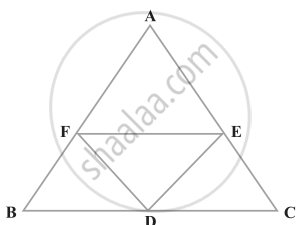Exercise 8.2 | Q 10 | Page 76

In figure, ABCD and AEFG are two parallelograms. If ∠C = 55º, determine ∠F.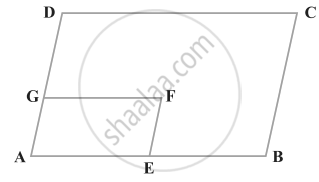Exercise 8.2 | Q 11 | Page 76

Exercise 8.2 | Q 12 | Page 76

Exercise 8.2 | Q 13 | Page 76

Diagonals of a quadrilateral ABCD bisect each other. If ∠A = 35º, determine ∠B.

Exercise 8.2 | Q 14 | Page 76

Opposite angles of a quadrilateral ABCD are equal. If AB = 4 cm, determine CD.

Exercise 8.3 [Pages 78 - 79]

### NCERT solutions for Mathematics Exemplar Class 9 Chapter 8 Quadrilaterals Exercise 8.3 [Pages 78 - 79]

Exercise 8.3 | Q 1 | Page 78

One angle of a quadrilateral is of 108º and the remaining three angles are equal. Find each of the three equal angles.

Exercise 8.3 | Q 2 | Page 78

ABCD is a trapezium in which AB || DC and ∠A = ∠B = 45º. Find angles C and D of the trapezium.

Exercise 8.3 | Q 3 | Page 78

The angle between two altitudes of a parallelogram through the vertex of an obtuse angle of the parallelogram is 60º. Find the angles of the parallelogram.

Exercise 8.3 | Q 4 | Page 78

ABCD is a rhombus in which altitude from D to side AB bisects AB. Find the angles of the rhombus.

Exercise 8.3 | Q 5 | Page 78

E and F are points on diagonal AC of a parallelogram ABCD such that AE = CF. Show that BFDE is a parallelogram.

Exercise 8.3 | Q 6 | Page 78

E is the mid-point of the side AD of the trapezium ABCD with AB || DC. A line through E drawn parallel to AB intersect BC at F. Show that F is the mid-point of BC.

Exercise 8.3 | Q 7 | Page 78

Through A, B and C, lines RQ, PR and QP have been drawn, respectively parallel to sides BC, CA and AB of a ∆ABC as shown in figure. Show that BC = 1/2 QR.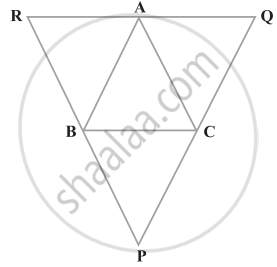Exercise 8.3 | Q 8 | Page 78

D, E and F are the mid-points of the sides BC, CA and AB, respectively of an equilateral triangle ABC. Show that ∆DEF is also an equilateral triangle.

Exercise 8.3 | Q 9 | Page 79

Points P and Q have been taken on opposite sides AB and CD, respectively of a parallelogram ABCD such that AP = CQ (figure). Show that AC and PQ bisect each other.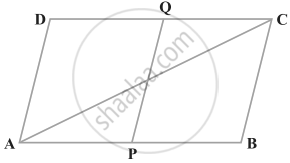Exercise 8.3 | Q 10 | Page 79

In figure, P is the mid-point of side BC of a parallelogram ABCD such that ∠BAP = ∠DAP. Prove that AD = 2CD.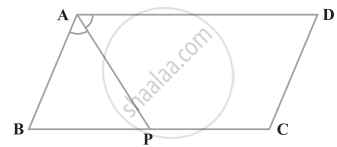Exercise 8.4 [Pages 82 - 83]

### NCERT solutions for Mathematics Exemplar Class 9 Chapter 8 Quadrilaterals Exercise 8.4 [Pages 82 - 83]

Exercise 8.4 | Q 1 | Page 82

A square is inscribed in an isosceles right triangle so that the square and the triangle have one angle common. Show that the vertex of the square opposite the vertex of the common angle bisects the hypotenuse.

Exercise 8.4 | Q 2 | Page 82

In a parallelogram ABCD, AB = 10 cm and AD = 6 cm. The bisector of ∠A meets DC in E. AE and BC produced meet at F. Find the length of CF.

Exercise 8.4 | Q 3 | Page 82

P, Q, R and S are respectively the mid-points of the sides AB, BC, CD and DA of a quadrilateral ABCD in which AC = BD. Prove that PQRS is a rhombus.

Exercise 8.4 | Q 4 | Page 82

P, Q, R and S are respectively the mid-points of the sides AB, BC, CD and DA of a quadrilateral ABCD such that AC ⊥ BD. Prove that PQRS is a rectangle.

Exercise 8.4 | Q 5 | Page 82

P, Q, R and S are respectively the mid-points of sides AB, BC, CD and DA of quadrilateral ABCD in which AC = BD and AC ⊥ BD. Prove that PQRS is a square.

Exercise 8.4 | Q 6 | Page 82

A diagonal of a parallelogram bisects one of its angles. Show that it is a rhombus.

Exercise 8.4 | Q 7 | Page 82

P and Q are the mid-points of the opposite sides AB and CD of a parallelogram ABCD. AQ intersects DP at S and BQ intersects CP at R. Show that PRQS is a parallelogram.

Exercise 8.4 | Q 8 | Page 82

ABCD is a quadrilateral in which AB || DC and AD = BC. Prove that ∠A = ∠B and ∠C = ∠D.

Exercise 8.4 | Q 9 | Page 82

In figure, AB || DE, AB = DE, AC || DF and AC = DF. Prove that BC || EF and BC = EF.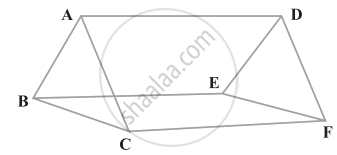Exercise 8.4 | Q 10 | Page 82

E is the mid-point of a median AD of ∆ABC and BE is produced to meet AC at F. Show that AF = 1/3AC

Exercise 8.4 | Q 11 | Page 82

Show that the quadrilateral formed by joining the mid-points of the consecutive sides of a square is also a square.

Exercise 8.4 | Q 12 | Page 83

E and F are respectively the mid-points of the non-parallel sides AD and BC of a trapezium ABCD. Prove that EF || AB and EF = 1/2 (AB + CD).

Exercise 8.4 | Q 13 | Page 83

Prove that the quadrilateral formed by the bisectors of the angles of a parallelogram is a rectangle.

Exercise 8.4 | Q 14 | Page 83

P and Q are points on opposite sides AD and BC of a parallelogram ABCD such that PQ passes through the point of intersection O of its diagonals AC and BD. Show that PQ is bisected at O.

Exercise 8.4 | Q 15 | Page 83

ABCD is a rectangle in which diagonal BD bisects ∠B. Show that ABCD is a square

Exercise 8.4 | Q 16 | Page 83

D, E and F are respectively the mid-points of the sides AB, BC and CA of a triangle ABC. Prove that by joining these mid-points D, E and F, the triangles ABC is divided into four congruent triangles.

Exercise 8.4 | Q 17 | Page 83

Prove that the line joining the mid-points of the diagonals of a trapezium is parallel to the parallel sides of the trapezium.

Exercise 8.4 | Q 18 | Page 83

P is the mid-point of the side CD of a parallelogram ABCD. A line through C parallel to PA intersects AB at Q and DA produced at R. Prove that DA = AR and CQ = QR.

Exercise 8.1Exercise 8.2Exercise 8.3Exercise 8.4## NCERT solutions for Mathematics Exemplar Class 9 chapter 8 - Quadrilaterals

NCERT solutions for Mathematics Exemplar Class 9 chapter 8 (Quadrilaterals) include all questions with solution and detail explanation. This will clear students doubts about any question and improve application skills while preparing for board exams. The detailed, step-by-step solutions will help you understand the concepts better and clear your confusions, if any. Shaalaa.com has the CBSE Mathematics Exemplar Class 9 solutions in a manner that help students grasp basic concepts better and faster.

Further, we at Shaalaa.com provide such solutions so that students can prepare for written exams. NCERT textbook solutions can be a core help for self-study and acts as a perfect self-help guidance for students.

Concepts covered in Mathematics Exemplar Class 9 chapter 8 Quadrilaterals are Concept of Quadrilaterals - Sides, Adjacent Sides, Opposite Sides, Angle, Adjacent Angles and Opposite Angles, Angle Sum Property of a Quadrilateral, Types of Quadrilaterals, Another Condition for a Quadrilateral to Be a Parallelogram, Theorem of Midpoints of Two Sides of a Triangle, Property: The Opposite Sides of a Parallelogram Are of Equal Length., Theorem: A Diagonal of a Parallelogram Divides It into Two Congruent Triangles., Theorem : If Each Pair of Opposite Sides of a Quadrilateral is Equal, Then It is a Parallelogram., Property: The Opposite Angles of a Parallelogram Are of Equal Measure., Theorem: If in a Quadrilateral, Each Pair of Opposite Angles is Equal, Then It is a Parallelogram., Property: The diagonals of a parallelogram bisect each other. (at the point of their intersection), Theorem : If the Diagonals of a Quadrilateral Bisect Each Other, Then It is a Parallelogram.

Using NCERT Class 9 solutions Quadrilaterals exercise by students are an easy way to prepare for the exams, as they involve solutions arranged chapter-wise also page wise. The questions involved in NCERT Solutions are important questions that can be asked in the final exam. Maximum students of CBSE Class 9 prefer NCERT Textbook Solutions to score more in exam.

Get the free view of chapter 8 Quadrilaterals Class 9 extra questions for Mathematics Exemplar Class 9 and can use Shaalaa.com to keep it handy for your exam preparation